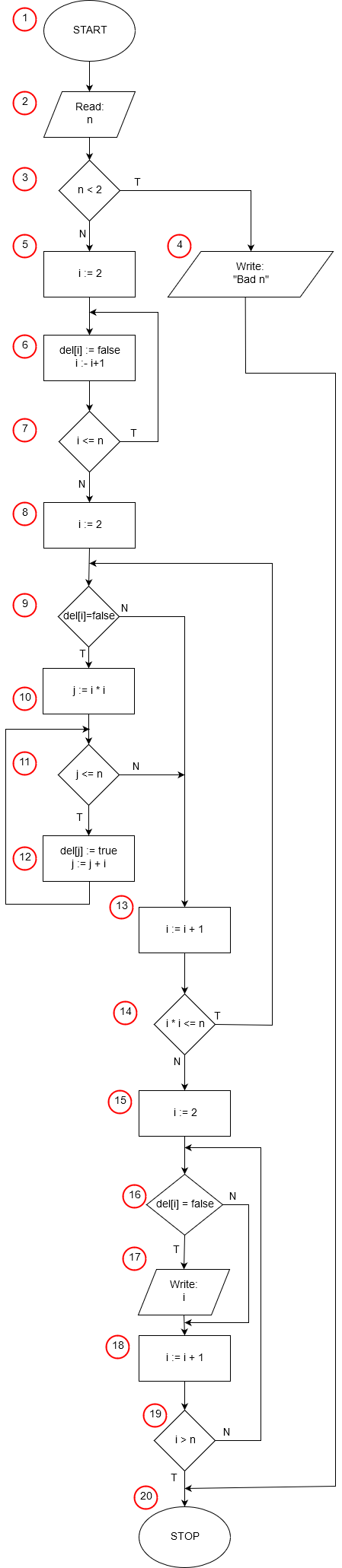Prime numbers - Eratosthenes sieve

Prime number is a natural number greater than 1 divided by 1 and itself (eg 2, 3, 5, 7, 11 ...). Already the ancient Greek mathematician and philosopher Eratosthenes noted that the above definition of natural numbers can be formulated slightly differently, namely that the prime number is a natural number that is not a multiple of two other natural numbers greater than 1. This observation became the basis of his The algorithm for finding all natural numbers in the range of 2 to any given integer n.

The idea of ​​the algorithm consists in deleting in the interval from 2 to n all multiples of consecutive numbers, Numbers that remain indefinite are not multiples of any other numbers in that range, so they are prime numbers.Implementation of the algorithm in: Pascal, C++, Java, Python, JavaScript

1. START - the beginning of the algorithm.
2. We load the upper value of the range in which we will look for prime numbers (from 2 to n).
3. We check that n is not less than 2. The smallest prime is 2.
4. If n is less than two we print out a message saying that there are no prime numbers smaller than 2 and we exit the algorithm, we go to point 20 - STOP.
5. If n has a valid value, we assign it to variable and value 2.
6. The element with the number and in the table del is set to false and then we increment the value of the variable i by 1. In the table del we store information whether the number of the given number was executed during the algorithm was deleted. Initially no number is deleted, so in points 5-7 we initialize all elements of the array with false values.
7. We check if and is less than or equal to the n - upper limit of the search. If the condition is satisfied, we return to point 6 of our algorithm to initialize the next item of the array.
8. When the condition from the previous point is not satisfied (and is greater than n) we have initialized the whole array skr and we can begin the essential part of our algorithm. At the beginning, we assign a variable and a value of 2, the first number whose multiple we will denote.
9. We are checking whether the number has been deleted and has not been deleted. If so, it means that all multiples have also been deleted. In this situation, we go to point 13 of the algorithm - we increase and by 1, ie we check the next number.
10. If the number has not been deleted so far, it means that it is the first number and, second, that all its multiples should be deleted. Notice that all multiples i below i * i have been deleted earlier. For example, for i=5 10 has already been deleted when plotting multiple 2, 15 was deleted when plotting multiple 3, 20 was plotted by plotting multiple 4. The first not deleted yet multiple of 5 will be 5*5: 25. Value i2 of the first non-dashed even multiples of the number i.
11. If the value of the numbering j and the multiple of the number i lie within the range in which we are looking for prime numbers (is less than or equal to n) we go to point 12, otherwise we go to point 13 of our algorithm - all multiples of the current value and are deleted and we can Increase the value of i.
12. In point 12 we find only if the next multiple of the number and contained in the variable j falls within the scope of the search for prime numbers. We denote the element of the number j in the array skr as deleted (del[j]: = true) and then increase the value of the variable j by i, j becomes another multiple of i. When the value j increases, we return to point 11.
13. We arrive at this place every time we plot all the multiple of the number i. We increase by 1 and we prepare ourselves to subtract the multiple of the next number.
14. In point 10 of the algorithm we have noticed that the smallest multiple of the number and which we have to plot is i2. At this point we check whether i * i <= n, that is, for the currently reached value and remain within the range of the unrelated multiple of i. If so, we return to point 9 of the algorithm - we continue to plot. Otherwise we find that all possible multiple of numbers from 2 to n have already been deleted - the numbers that remain underscored are prime numbers. In subsequent points of the algorithm we will write them on the screen.
15. We need to check the values ​​of all the elements of the array skr in turn and print out the numbers that were not deleted (del[i] is false). The next elements will be numbered variable i, for this purpose, we initialize it with value 2 - the number of the first element of our array.
16. We check whether the number i remain unnoticed. If the condition is not fulfilled, that is, the number is skipped, jump to point 18 of the algorithm by jumping out the number 17 in the prime number.
17. If the number i remain unspecified we print its value - the value of the variable i, for which the element of the array del[i] is false.
18. We increment by 1 the value of the variable i go to the next number.
19. We look at whether we have already checked all numbers in the range 2 to n. If we do not return to point 16.
20. STOP - the end of the algorithm.